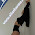Today we will start here with the concept of spring index with the help of this post. Further, we will derive the expression for stresses and also deflections developed in various types of springs.

Let us go ahead step by step for easy understanding, however if there is any issue we can discuss it in comment box which is provided below this post.

### So, what is a spring?

Spring is basically defined as the elastic element which is basically used for absorbing the energy due to resilience and this absorbed energy may be released as and when required. For detailed post about the concepts and types of springs, we may find our recent post i.e. spring in strength of material.

### Spring index

Spring index provides a relation between mean diameter of spring and wire diameter of spring. Spring index is basically defined as the ratio of mean diameter of spring to the wire diameter of spring.

We have used one word here related with spring i.e. mean diameter of spring. Therefore, we must have to understand here the mean diameter of spring.

Mean diameter of spring could be determined by subtracting the wire diameter of spring from outer diameter of spring.
When mean diameter of spring will be divided by the wire diameter of spring, we will have the value of spring index.

Spring index will be indicated by C and as spring index is basically one ratio, hence spring index will be unit less.
Spring index value will be in the range of 4 to 10.

Do you have suggestions? Please write in comment box.
We will now see various terminologies of springs, in the category of strength of material, in our next post.

### Reference:

Strength of material, By R. K. Bansal

1.2.